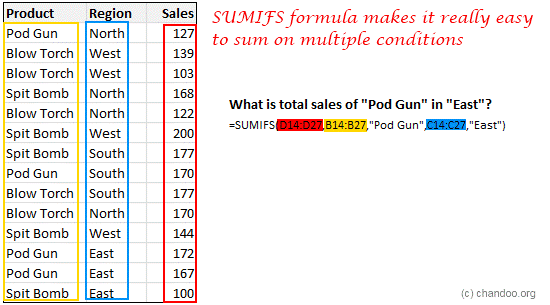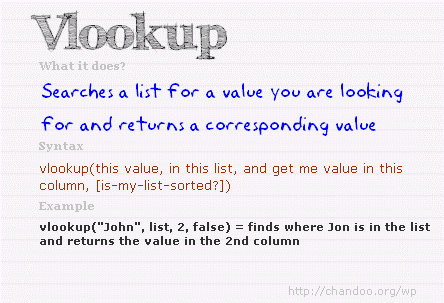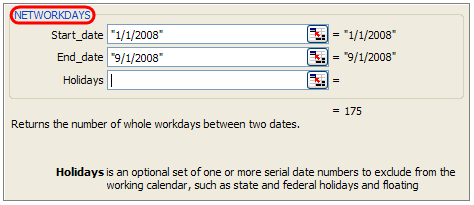# Top Formulas for AnalystsIf you are wondering what the top ten formulas are, then you should really consider acknowledging the fact that there is no standard list of the perfect ten formulas for each individual. However, you will need to keep the following formulas in mind if you are a developing business analyst. You must make yourself familiar with the following ten formulas:

This formula will make it simple to add up different conditions such as the number of products sold in region A or the most popular customer type C in the month of M. In order to filter all of this information in an organized manner, you can make use of the SUMIFS formula, which is capable of summing up a set of data that meet different conditions. The syntax of this formula is =SUMIFS(the data that you would like to add up, condition column#1, condition, condition column#2, condition...).This formula is very helpful because it assists the users search for data in a set of numbers and gives the user a corresponding value. For example, if you are searching for the name of a client whose ID number is ID=C00078 or the price of a product that has a code of =p0013, then VLOOKUP is what you need. The syntax of this formula is very simple to remember =VLOOKUP(what you are searching for, column from what you would like to search the result).While VLOOKUP will assist you in searching for data, sometimes it might not be able to provide you with every single piece of information that you are looking for because they might be in different columns. For example, if you are searching for the customer name in the left column and their ID in the right column, then you will only be able to discover their name because VLOOKUP only works in the left most columns. However, Index+Match formula will help you look through any column and provide a corresponding value from other sets of data in surrounding columns as well. The syntax of this formula is =INDEX(customer name, MATCH(C00045”, customer ID, 0) ).

• IF Formula

The IF formula helps you make decisions. For example, if your company is considering giving a 20% pay hike to every person who visits a particular website and 10% hike to the rest, how would you display this in an Excel file? You would follow the IF formula syntax which is IF(condition to text, output for TRUE, output for FALSE).

• Nesting Formula

To take care of the more complex decisions, businesses have to make use of the Nesting formula. Nesting generally means to include one formula within another formula. This basically means nesting one formula within another formula. The syntax would remain the same, and you can nest any formula within another formula as many times as you like.

• Basic Arithmetic

The basic arithmetic functions have been taught to everyone in school, however if you do not remember them, then a few operators have been listed below to help you revive what basic arithmetic is all about.

• +, -, *, / These are the basic operators of addition, subtraction, multiplication and division

• ^ This is to raise a number to the power of the value of another number.

• ( ) Parenthesis must be solved first in any equation or calculation

• & This is to combine two values

• % The percent sign tells you to divide by 100

These were just a few of the basic arithmetic operators you will come across while using Excel. You can explore the other basic functions in your Excel window.

The following TEXT formulas must be kept in mind if you are an aspiring analyst.

• Substitute: This will replace the text.

• Text: You can use this formula to convert a value to text format.

• Len: This helps to evaluate the length of a piece of text.

• Trim: This will assist you in removing any unnecessary spaces from the test.

• Left, Right, Mid: You can take out portion of the text from these areas.

• Find: You can search for text with this formula.

• Workday and Networkdays Formulas

Whether there are enough days in the week or not, you must learn to work with the everyday calculations. For example, if you are beginning a project that will take around one hundred and eighty workdays to finish up and you need to begin on January 16, what would the ending date of the project be? In order to figure this out, you can utilize the WORKDAY formula of Excel and find out.

Similarly, NETWORKDAYS formulas inform us about how many working days there are between a set of dates.Both of these formulas will allow you to take holidays and vacations into consideration as well, such as:

• NETWORKDAYS.INTL: This formula will let you customize the weekends.

• NETWORKDAYS: This formula will calculate the number of days that you will be working between a set of dates.

• WORKDAY.INTL: This formula will let you customize the weekends.

• WORKDAY: This formula will calculate the ending date from the starting date of a task.

• SMALL and LARGE Formulas

The SMALL and LARGE formulas are a very important aspect when it comes to business in the following ways:

• SMALL: This formula will help you finding the smallest value from the list of data.

• LARGE: This formula is to spot out the largest value from the list.

• MIN: This formula will give the minimum value present on the list.

• MAX: The MAX formula will provide you with the maximum value.

• RANK: This formula assists in ranking the values in order from a list.

- IFERROR Formula

This formula will assist you in correcting any errors present in your formula and prevent you from making mistakes while conducting your tasks.